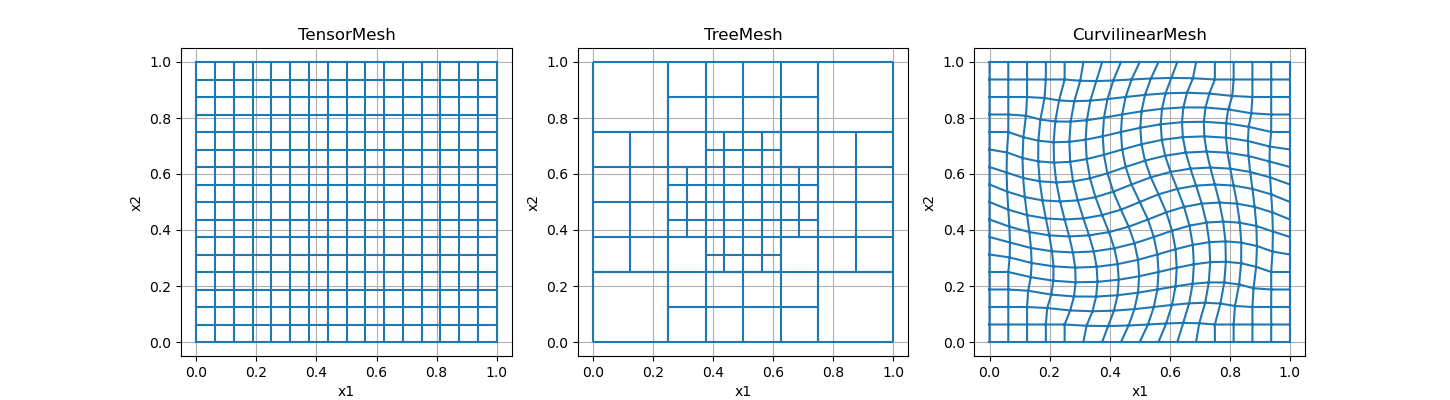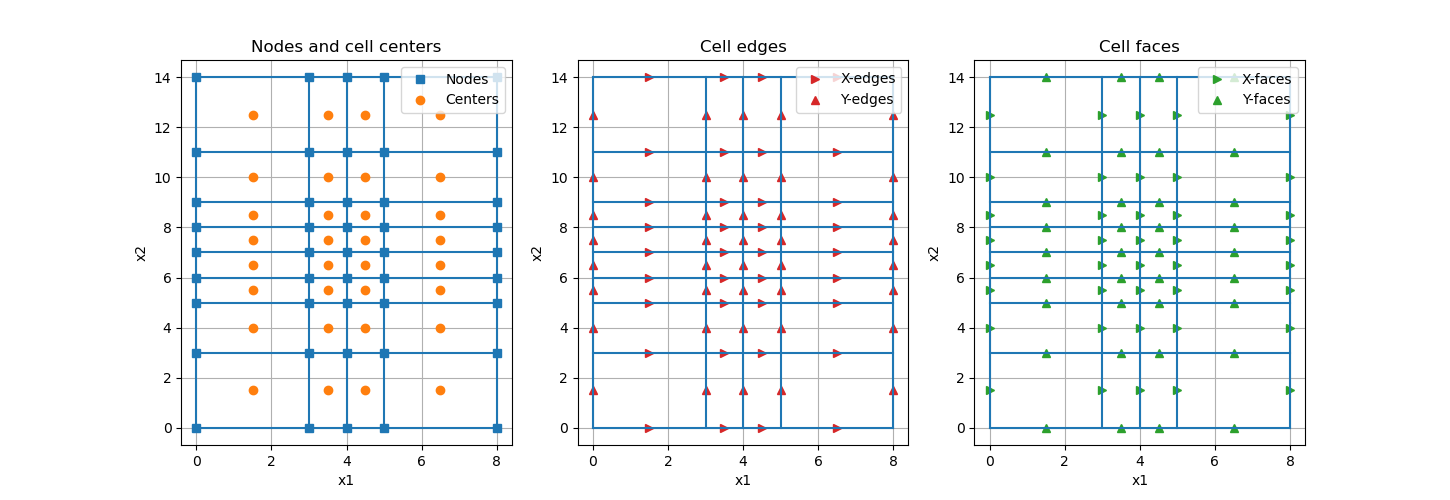# Overview of Mesh Types#

Here we provide an overview of mesh types and define some terminology. Separate tutorials have been provided for each mesh type.

```import numpy as np
import discretize
import matplotlib.pyplot as plt
```

## General Categories of Meshes#

The three main types of meshes in discretize are

Examples for each mesh type are shown below.

```ncx = 16  # number of cells in the x-direction
ncy = 16  # number of cells in the y-direction

# create a tensor mesh
tensor_mesh = discretize.TensorMesh([ncx, ncy])

# create a tree mesh and refine some of the cells
tree_mesh = discretize.TreeMesh([ncx, ncy])

def refine(cell):
if np.sqrt(((np.r_[cell.center] - 0.5) ** 2).sum()) < 0.2:
return 4
return 2

tree_mesh.refine(refine)

# create a curvilinear mesh
curvi_mesh = discretize.CurvilinearMesh(
discretize.utils.exampleLrmGrid([ncx, ncy], "rotate")
)

# Plot
fig, axes = plt.subplots(1, 3, figsize=(14.5, 4))
tensor_mesh.plotGrid(ax=axes)
axes.set_title("TensorMesh")

tree_mesh.plotGrid(ax=axes)
axes.set_title("TreeMesh")

curvi_mesh.plotGrid(ax=axes)
axes.set_title("CurvilinearMesh")
``````Text(0.5, 1.0, 'CurvilinearMesh')
```

## Variable Locations and Terminology#

When solving differential equations on a numerical grid, variables can be defined on:

• nodes

• cell centers

• cell faces

• cell edges

Below we show an example for a 2D tensor mesh.

```hx = np.r_[3, 1, 1, 3]
hy = np.r_[3, 2, 1, 1, 1, 1, 2, 3]
tensor_mesh2 = discretize.TensorMesh([hx, hy])

# Plot
fig, axes2 = plt.subplots(1, 3, figsize=(14.5, 5))
tensor_mesh2.plotGrid(ax=axes2, nodes=True, centers=True)
axes2.legend(("Nodes", "Centers"))
axes2.set_title("Nodes and cell centers")

tensor_mesh2.plotGrid(ax=axes2, edges=True)
axes2.legend(("X-edges", "Y-edges"))
axes2.set_title("Cell edges")

tensor_mesh2.plotGrid(ax=axes2, faces=True)
axes2.legend(("X-faces", "Y-faces"))
axes2.set_title("Cell faces")
``````Text(0.5, 1.0, 'Cell faces')
```

Note that we define X-edges as being edges that lie parallel to the x-axis. And we define X-faces as being faces whose normal lies parallel to the axis. In 3D, the difference between edges and faces is more obvious.

Total running time of the script: ( 0 minutes 0.355 seconds)

Gallery generated by Sphinx-Gallery